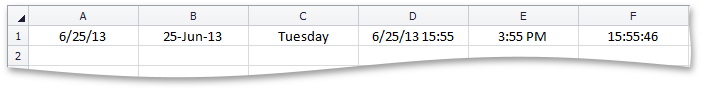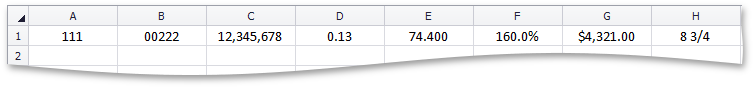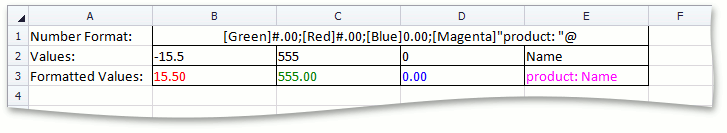# How to: Specify Number or Date Format for Cell Content

The Spreadsheet control uses Microsoft Excel number formats to display cell numbers as percentage, decimal, currency, accounting, and date/time values. To apply a number format to a cell or cell range, assign an appropriate format code to the NumberFormat or NumberFormatLocal property. This topic contains examples that demonstrate how to use standard and custom number formats to format numeric cell values.

## Date and Time Formats

This example demonstrates how to specify different display formats for date and time values in cells.

``````worksheet.Range["A1:F1"].Formula = "= Now()";

// Apply different date display formats.
worksheet.Cells["A1"].NumberFormat = "m/d/yy";

worksheet.Cells["B1"].NumberFormat = "d-mmm-yy";

worksheet.Cells["C1"].NumberFormat = "dddd";

// Apply different time display formats.
worksheet.Cells["D1"].NumberFormat = "m/d/yy h:mm";

worksheet.Cells["E1"].NumberFormat = "h:mm AM/PM";

worksheet.Cells["F1"].NumberFormat = "h:mm:ss";
``````

The image below shows different formats used to display date and time values in cells.## Number Formats

This example demonstrates how to use different number formats to display numeric values in cells.

``````// Display 111 as 111.
worksheet.Cells["A1"].Value = 111;
worksheet.Cells["A1"].NumberFormat = "#####";

// Display 222 as 00222.
worksheet.Cells["B1"].Value = 222;
worksheet.Cells["B1"].NumberFormat = "00000";

// Display 12345678 as 12,345,678.
worksheet.Cells["C1"].Value = 12345678;
worksheet.Cells["C1"].NumberFormat = "#,#";

// Display .126 as 0.13.
worksheet.Cells["D1"].Value = .126;
worksheet.Cells["D1"].NumberFormat = "0.##";

// Display 74.4 as 74.400.
worksheet.Cells["E1"].Value = 74.4;
worksheet.Cells["E1"].NumberFormat = "##.000";

// Display 1.6 as 160.0%.
worksheet.Cells["F1"].Value = 1.6;
worksheet.Cells["F1"].NumberFormat = "0.0%";

// Display 4321 as \$4,321.00.
worksheet.Cells["G1"].Value = 4321;
worksheet.Cells["G1"].NumberFormat = "\$#,##0.00";

// Display 8.75 as 8 3/4.
worksheet.Cells["H1"].Value = 8.75;
worksheet.Cells["H1"].NumberFormat = "# ?/?";
``````

The image below shows different formats used to display cell numbers.## Custom Number Formats

This example demonstrates how to create a custom number format that uses different formats and colors to display positive numbers, negative numbers, zero values, and text. The code sections are separated by semicolons and used in the following order:

<POSITIVE>;<NEGATIVE>;<ZERO>;<TEXT>

Refer to the following topic for more information on how to create a custom number format: Number format codes.

``````// Set cell values.
worksheet["B2:B3"].Value = -15.50;
worksheet["C2:C3"].Value = 555;
worksheet["D2:D3"].Value = 0;
worksheet["E2:E3"].Value = "Name";

// Apply custom number format.
worksheet["B3:E3"].NumberFormat = "[Green]#.00;[Red]#.00;[Blue]0.00;[Magenta]\"product: \"@";
``````

The image below shows how different values are displayed in cells when a custom number format is applied.